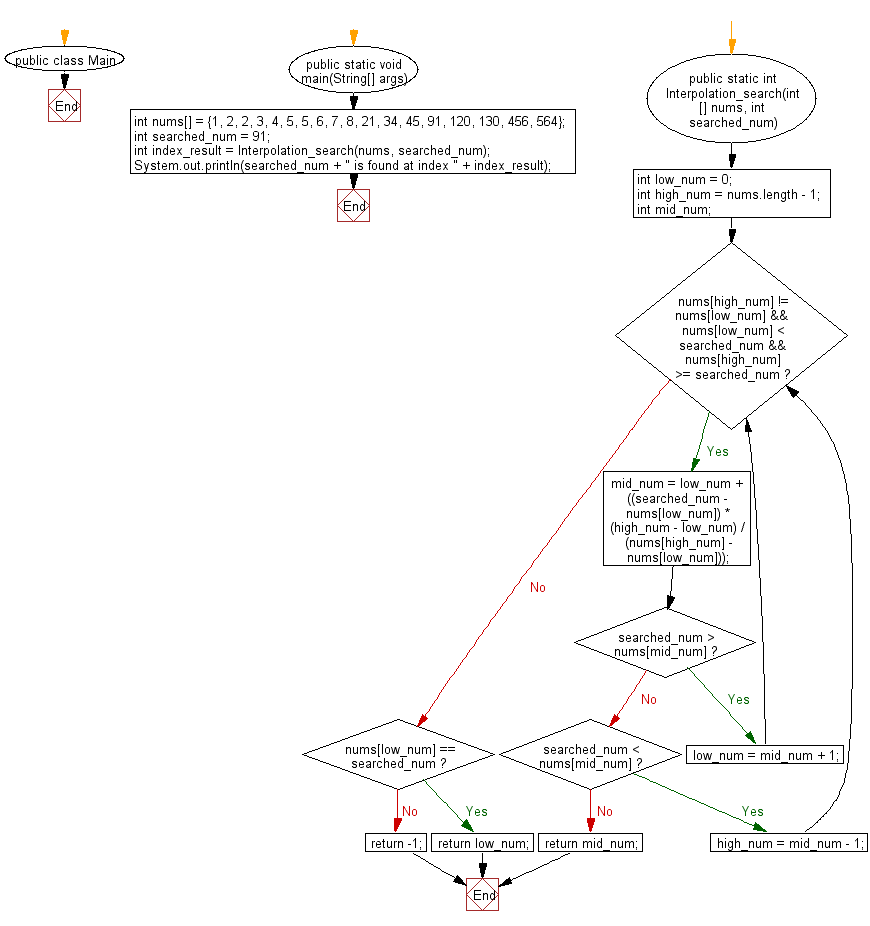﻿ Java exercises: Find a specified element in a given array of elements using Interpolation Search - w3resource# Java Exercises: Find a specified element in a given array of elements using Interpolation Search

## Java Search: Exercise-4 with Solution

Write a Java program to find a specified element in a given array of elements using Interpolation Search.

From Wikipedia, Interpolation search is an algorithm for searching for a key in an array that has been ordered by numerical values assigned to the keys (key values). It was first described by W. W. Peterson in 1957. Interpolation search resembles the method by which people search a telephone directory for a name (the key value by which the book's entries are ordered): in each step the algorithm calculates where in the remaining search space the sought item might be, based on the key values at the bounds of the search space and the value of the sought key, usually via a linear interpolation. The key value actually found at this estimated position is then compared to the key value being sought. If it is not equal, then depending on the comparison, the remaining search space is reduced to the part before or after the estimated position. This method will only work if calculations on the size of differences between key values are sensible.

Sample Solution:

Java Code:

``````public class Main {

public static void main(String[] args){
int nums[] = {1, 2, 2, 3, 4, 5, 5, 6, 7, 8, 21, 34, 45, 91, 120, 130, 456, 564};
int searched_num = 91;
// Find the index of searched item
int index_result = Interpolation_search(nums, searched_num);
System.out.println(searched_num + " is found at index " + index_result);
}

public static int Interpolation_search(int[] nums, int searched_num) {
int low_num = 0;
int high_num = nums.length - 1;
int mid_num;
while (nums[high_num] != nums[low_num] && nums[low_num] < searched_num && nums[high_num] >= searched_num) {
mid_num = low_num + ((searched_num - nums[low_num]) * (high_num - low_num) / (nums[high_num] - nums[low_num]));
if (searched_num > nums[mid_num])
low_num = mid_num + 1;
else if (searched_num < nums[mid_num])
high_num = mid_num - 1;
else
return mid_num;
}
if (nums[low_num] == searched_num) {
return low_num;
} else {
return -1;
}
}
}
```
```

Sample Output:

```91 is found at index 13
```

Flowchart:Java Code Editor:

What is the difficulty level of this exercise?

﻿

## Java: Tips of the Day

Array vs ArrayLists:

The main difference between these two is that an Array is of fixed size so once you have created an Array you cannot change it but the ArrayList is not of fixed size. You can create instances of ArrayLists without specifying its size. So if you create such instances of an ArrayList without specifying its size Java will create an instance of an ArrayList of default size.

Once an ArrayList is full it re-sizes itself. In fact, an ArrayList is internally supported by an array. So when an ArrayList is resized it will slow down its performance a bit as the contents of the old Array must be copied to a new Array.

At the same time, it's compulsory to specify the size of an Array directly or indirectly while creating it. And also Arrays can store both primitives and objects while ArrayLists only can store objects.

Ref: https://bit.ly/3o8L2KH